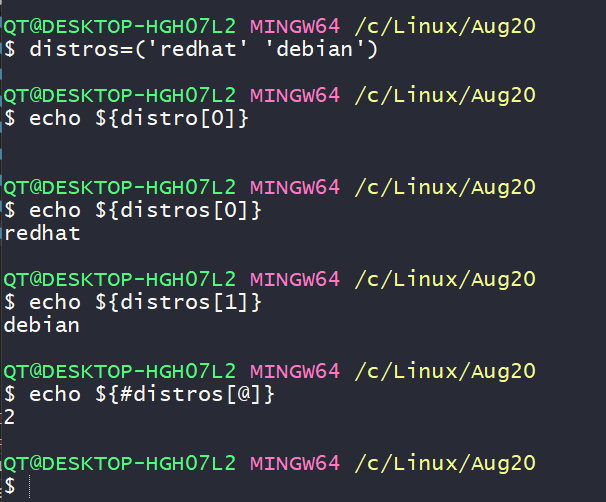### How to pass arrays to the functions

• Lets pass arguments to shell script which in turn passes array to the function
``````#!/bin/bash
display_array() {
array=\$@
echo "The array inside the function is \${array[*]}"
for item in "\$@"
do
echo "Argument is \${item}"
done
}

test_input_1=(1 2 3 4 5)

for item in "\${test_input_1[@]}"
do
echo "External \$item"
done

echo "The orginal array for test input 1 is \${test_input_1[*]}"
echo "Calling function"
display_array \${test_input_1[*]}

``````
• Execute the following commands as shown below### Variable scope

• By default variables inside the function are global variables
``````#!/bin/bash

test_variable_1=10

testfunction() {
test_variable_1=50
test_variable_2=100
}

testfunction
echo "\$test_variable_1"
echo "\$test_variable_2"
``````
• In shell if your variable has to be local inside function, you have to explicit
``````#!/bin/bash

test_variable_1=10

testfunction() {
test_variable_1=50
test_variable_2=100
local test_variable_3=200
echo "Inside function: \$test_variable_3"
}

testfunction
echo "\$test_variable_1"
echo "\$test_variable_2"
echo "Outside function: \$test_variable_3"
``````

### Returning values from functions

• Shell sample
``````#!/bin/bash
print_message() {
input="\$1"
output="Message is \${input}"
return \$output
}